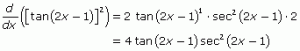# Problem 11: Chain Rule

2011-2012 Chain Rule Problems Calculate the derivative of tan2(2x –1) with respect tox using the chain rule, and then verify your answer using a second differentiation technique. Solution for Chain Rule Practice Problems: Note that tan2(2x –1) = [tan (2x – 1)]2. To find the solution for chain rule problems, complete these steps: Apply the power rule, … Continue reading "Problem 11: Chain Rule"##2011-2012 Chain Rule Problems

Calculate the derivative of tan2(2x –1) with respect tox using the chain rule, and then verify your answer using a second differentiation technique.

## Solution for Chain Rule Practice Problems:

Note that tan2(2x –1) = [tan (2x – 1)]2. To find the solution for chain rule problems, complete these steps:

1. Apply the power rule, changing the exponent of 2 into the coefficient of tan (2x – 1), and then subtracting 1 from the square.
2. Multiply by the expression tan (2x – 1), which was originally raised to the second power.
3. Take the derivative of tan (2x – 1) with respect to x.
4. Multiply by the derivative of 2x – 1, the expression that is plugged into tangent.

## Derivative of tan2x

These four steps are implemented in the solution below for derivative of tan2x.To verify the derivative, apply the product rule, noting that  tan2(2x –1) = tan (2x –1) · tan (2x – 1). Evev the product rule will require the chain rule, when you differentiate each factor (2x – 1), as demonstrated below.Both techniques for these chain rule practice problems result in the same derivative.

The video may take a few seconds to load.Having trouble Viewing Video content? Some browsers do not support this version – Try a different browser.